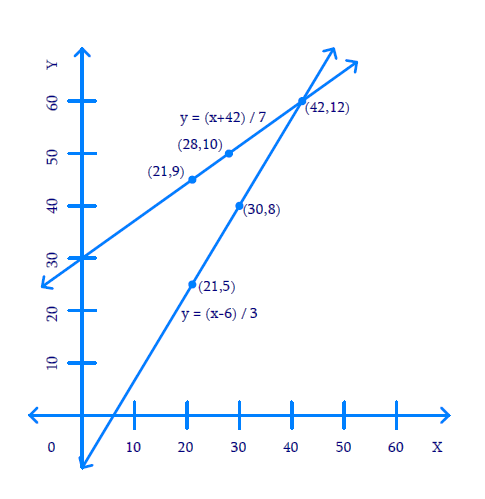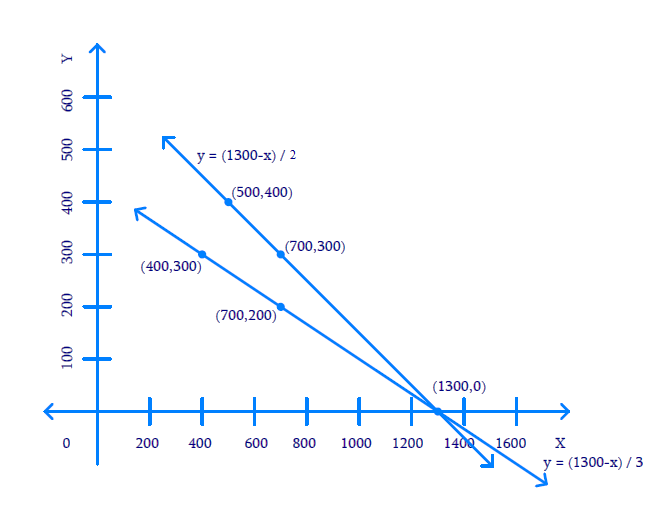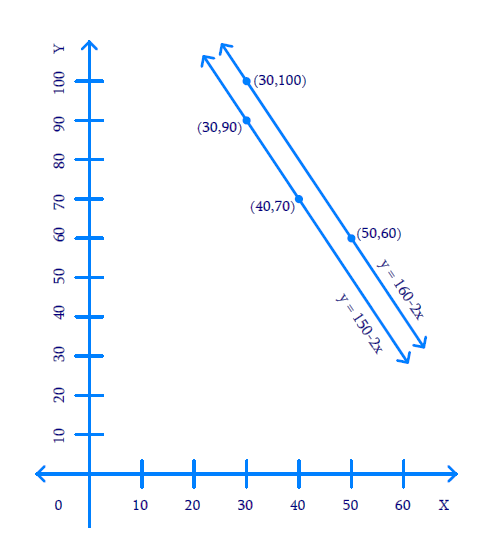# NCERT Solutions For Class 10 Maths Chapter 3 Exercise 3.1

Go back to  'Pair of Linear Equations in Two Variables'

## Chapter 3 Ex.3.1 Question 1

Aftab tells his daughter, “Seven years ago, I was seven times as old as you were then. Also, three years from now, I shall be three times as old as you will be.” (Isn’t this interesting?) Represent this situation algebraically and graphically.

### Solution

What is Known?

$$7$$ years ago, Aftab was $$7$$ times as old as his daughter then and $$3$$ years from now, Aftab shall be $$3$$ times as old as his daughter will be.

What is Unknown?

Represent the situation algebraically and graphically.

Reasoning:

Assume the present age of Aftab be $$x$$  years and his daughter be $$y$$ years then represent their ages $$7$$ years later and $$3$$ years ago in term of $$x$$ and $$y.$$ Two linear equations can be formed to represent the above situation algebraically.

Using algebraic equation and truth table they can be graphically represented.

Steps:

(i) Present age of Aftab $$= x$$ years and his daughter $$= y$$ years

Therefore, $$7$$ years ago, age of Aftab $$= \left( {x - 7} \right)$$ years and his daughter $$= \left( {y - 7} \right)$$years

Using this information and applying the known condition that $$7$$ years ago, Aftab was $$7$$ times as old as his daughter then:

\begin{align}x - 7 &= 7(y - 7)\\x - 7 &= 7y - 49\\x - 7y - 7 + 49 &= 0\\x - 7y + 42 &= 0\end{align}

After $$3$$ years from now, age of Aftab $$= \left( {x + 3} \right)$$ years and his daughter $$= \left( {y + 3} \right)$$ years and also Aftab will be $$3$$ times as old as his daughter. Then mathematically,

\begin{align}x + 3 &= 3\left( {y + 3} \right)\\x + 3 &= 3y + 9\\x - 3y + 3 - 9 &= 0\\x - 3y - 6 &= 0\end{align}

Algebraic representations, where $$x$$ and $$y$$ are present ages of Aftab and his daughter respectively:

\begin{align}x - 7y + 42 &= 0\qquad(1)\\x - 3y-6 &= 0\qquad(2)\end{align}

Therefore, the algebraic representation is for equation $$1$$ is:

\begin{align}x - 7y + 42 &= 0\\- 7y &= - x - 42\\7y &= x + 42\\y &= \frac{{x + 42}}{7}\end{align}

And, algebraic representation for equation $$(2)$$ is:

\begin{align}x - 3y - 6 &= 0\\- 3y &= - x + 6\\3y &= x - 6\\y &= \frac{{x - 6}}{3}\end{align}

Let us represent these equations graphically. For this, we need at least two solutions for each equation. We give these solutions in table shown below.

For equation $$(1)$$

 $$x$$ $$21$$ $$28$$ $$y = \frac{{x + 42}}{7}$$ $$9$$ $$10$$

For equation (2)

 $$x$$ $$30$$ $$21$$ $$y = \frac{{x - 6}}{3}$$ $$8$$ $$5$$

The graphical representation is as follows.Unit: $$1 \,\rm{cm}=5$$ years.

The answer is $$(42, \;12)$$

## Chapter 3 Ex.3.1 Question 2

The coach of a cricket team buys $$3$$ bats and 6 balls for $$₹\,3900.$$ Later, she buys another bat and $$3$$ more balls of the same kind for ₹ $$1300.$$ Represent this situation algebraically and geometrically.

### Solution

What is Known?

(i) Three bats and six balls for ₹ $$3900$$

(ii) One bat and three balls for ₹ $$1300$$

What is Unknown?

Represent the situation geometrically and algebraically

Reasoning:

Assuming the cost of one bat as ₹ $$x$$ and the cost of one ball as ₹ $$y,$$ two linear equations can be formed for the above situation.

Steps:

The cost of $$3$$ bats and 6 balls is ₹ $$3900.$$

Mathematically:

\begin{align}3x + 6y&= 3900\\3(x + 2y)& = 3900\\x + 2y& = 1300\end{align}

Also, the cost of $$1$$ bat and $$3$$ balls is ₹ $$1300.$$

Mathematically:

$x + 3y = 1300$

Algebraic representation where $$x$$ and $$y$$ are cost of bat and ball respectively.

\begin{align}x + 2y &= 1300 \qquad(1)\\x + 3y &= 1300\qquad(2)\end{align}

Therefore, the algebraic representation for equation $$1$$ is:

\begin{align}x + 2y &= 1300\\2y &= 1300-x\\y &= \frac{{1300 - x}}{2}\end{align}

And, the algebraic representation for equation $$2$$ is:

\begin{align}x + 3y &= 1300\\3y &= 1300-x\\y &= \frac{{1300 - x}}{3}\end{align}

Let us represent these equations graphically. For this, we need at least two solutions for each equation. We give these solutions in table shown below.

 $$x$$ $$700$$ $$500$$ $$y = \frac{{1300 - x}}{2}$$ $$300$$ $$400$$

 $$x$$ $$400$$ $$700$$ $$y = \frac{{1300 - x}}{3}$$ $$300$$ $$200$$

The graphical representation is as follows.Unit: $$1\,\rm{cm} =$$$$100.$$

The answer is $$(1300, \,0)$$

## Chapter 3 Ex.3.1 Question 3

The cost of $$2 \,\rm{kg}$$ of apples and $$1 \,\rm{kg}$$ of grapes on a day was found to be ₹ $$160$$ After a month, the cost of $$4\,\rm{kg}$$ of apples and $$2\,\rm{kg}$$ of grapes is ₹ $$300$$ Represent the situation algebraically and geometrically.

### Solution

What is Known?

(i) Cost of $$2 \,\rm{kg}$$of apples and $$1 \,\rm{kg}$$ of grapes is ₹ $$160$$

(ii) Cost of $$4 \,\rm{kg}$$ of apples and $$2 \,\rm{kg}$$ of grapes is ₹ $$300.$$

What is Unknown?

Represent the situation geometrically and algebraically.

Reasoning:

Assuming the cost of $$1 \,\rm{kg}$$ apples as ₹ $$x$$ and the cost of $$1 \,\rm{kg}$$ grapes as ₹ $$y,$$ two linear equations can be formed for the above situation.

Steps:

Let the cost of $$1 \,\rm{kg}$$ of apples be $$x$$ and cost of $$1 \,\rm{kg}$$ of grapes be $$y$$

Cost kg $$2 \,\rm{kg}$$ apples and $$1 \,\rm{kg}$$ of grapes is ₹ $$160.$$

Mathematically,

$2x + y = 160$

Also, cost kg $$4 \,\rm{kg}$$ apples and $$2 \,\rm{kg}$$ of grapes is ₹ $$300.$$

Mathematically,

\begin{align}4x + 2y &= 300\\2(2x + y) &= 300\\2x\, + \,y\,& = \,150\end{align}

Algebraic representation where $$x$$ and $$y$$ are the cost of $$1 \,\rm{kg}$$ apple and $$1 \,\rm{kg}$$ grapes respectively.

\begin{align}2x + y &= 160 \,\dots(1)\\2x + y& = 150\,\dots(2)\end{align}

Therefore, the algebraic representation is for equation $$1$$ is:

\begin{align}2x + y &= 160\\y &= 160-2x\end{align}

And, the algebraic representation is for equation $$2$$ is:

\begin{align}2x + y& = 150\\y &= 150-2x\end{align}

Let us represent these equations graphically. For this, we need at least two solutions for each equation. We give these solutions in table shown below.

 $$x$$ $$50$$ $$30$$ $$y = 160-2x$$ $$60$$ $$100$$

 $$x$$ $$30$$ $$40$$ $$y = 150-2x$$ $$90$$ $$70$$

The graphical representation is as follows.Unit $$= 1\, \rm{cm} =$$$$10$$

Since the lines are parallel hence no Solution